# Electric Potential Practice Level - 1

## 25 Questions MCQ Test Exclusive Video Lectures of Class 12 Physics by Experts | Electric Potential Practice Level - 1

Description
This mock test of Electric Potential Practice Level - 1 for Class 12 helps you for every Class 12 entrance exam. This contains 25 Multiple Choice Questions for Class 12 Electric Potential Practice Level - 1 (mcq) to study with solutions a complete question bank. The solved questions answers in this Electric Potential Practice Level - 1 quiz give you a good mix of easy questions and tough questions. Class 12 students definitely take this Electric Potential Practice Level - 1 exercise for a better result in the exam. You can find other Electric Potential Practice Level - 1 extra questions, long questions & short questions for Class 12 on EduRev as well by searching above.
QUESTION: 1

Solution:
QUESTION: 2

Solution:
QUESTION: 3

### Two point charges 4 μC and -2 μC are separated by a distance of 1 m in air. At what point in between the charges and on the line joining the charges, is the electric potential zero?

Solution:
QUESTION: 4

Three charges 2q, -q and -q are located at the vertices of an equilateral triangle. At the centre of the triangle

Solution:
QUESTION: 5

The veriation of potential with distance R from fixed point is shown in Fig.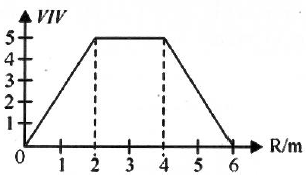The elecrtic field at R = 5 m is

Solution:
QUESTION: 6

When a 2 μC of charge is carried from point A to point B. the amount of work done by electric field is 50 μJ. What is the potential difference and which point is at a higher potential?

Solution:
QUESTION: 7

The work done in taking a unit positive charge from P to A is WA and from P to B is WB.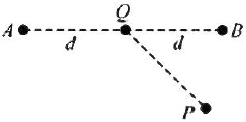Then

Solution:
QUESTION: 8

Four charges +q, -q, +q and -q are put together at four corners of a square as shown in Fig. The work done by external agent in assembling this configuration is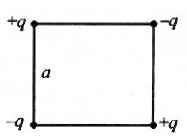Solution:
QUESTION: 9

The electric field in a region surrounding the origin is uniform and along the x-axis. A small circle is drawn with the centre at the origin cutting the axes at points A, B, C and D having coordinates (a, 0), (0, a), (-a, 0) and (0, -a), respectively as shown in Fig. Then, the potential is minimum at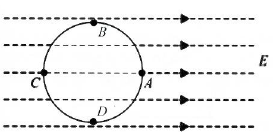Solution:
QUESTION: 10

Mark the correct statement.

Solution:
QUESTION: 11

Variation in potential is maximum if one goes

Solution:
QUESTION: 12

There is an electric field E in the x-direction. If the work done by electric field in moving a charge of 0.2 C through a distance of 2 m along a line making an angle 600 with the x-axis is 4 J, then what is the value of E?

Solution:
QUESTION: 13

Some equipotential surfaces are shown in Fig. The magnitude and direction of the electric field is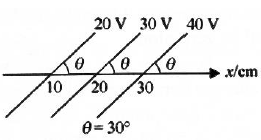Solution:
QUESTION: 14

In moving from A to B along an electric field line, the work done by the electric field on an electron is 6.4 × 10-19 J. If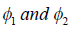equipotential surfaces, then the potential difference VC - VA is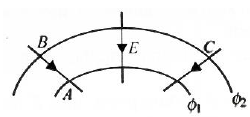Solution:
QUESTION: 15

An electron is taken from point A to point B along the path AB in a uniform electric field of intensity E = 10 Vm-1, Side AB = 5 m and side BC = 3 m. Then, the amount of work done on electron by us is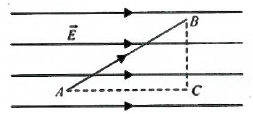Solution:
QUESTION: 16

Two identical rings P and Q of radius 0.1 m are mounted coaxially at a distance 0.5 m apart. The charges on the two rings are 2 and 4 μC respectively. The work done in transferring a charge of 5 μC from the centre of P to that of Q is

Solution:
QUESTION: 17

At a point in space, the electric field points towards north. In the region surrounding this point, the rate of change of potential will be zero along

Solution:
QUESTION: 18

Charges -q, Q and -q are placed at an equal distance on straight line. If the total potential energy of the system of three charges is zero, then find the ratio Q/q.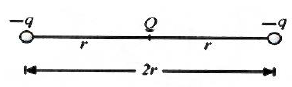Solution:
QUESTION: 19

Four identical charges are placed at the point (1, 0, 0 ), (0, 1 ,0), (-1, 0, 0) and (0, -1, 0). Then,

Solution:
QUESTION: 20

When the separation between two charges is increased, the electric potential energy of the charges

Solution:
QUESTION: 21

A positive charge is moved from a low potential point A to a high potential point B. Then the electric potential energy of the system

Solution:
QUESTION: 22

If a charge is moved against the coulomb force of an electric field, then the

Solution:
QUESTION: 23

Mark the correct statement:

Solution:
QUESTION: 24

The electric potential decreases uniformly from 120 V to 80 V as one moves on the X-axis from x = -1 cm to x = +1 cm. The electric field at the origin.

Solution:
QUESTION: 25

Charge Q is given a displacement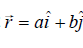in an electric field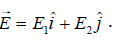The work done is

Solution: